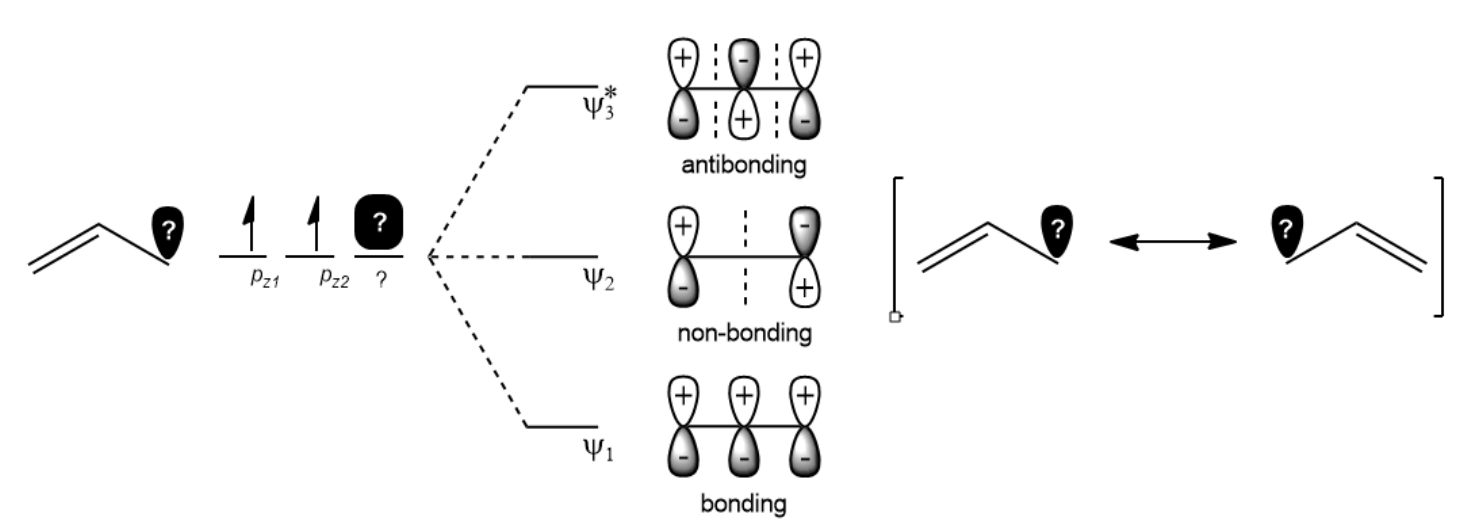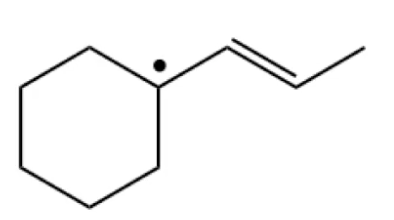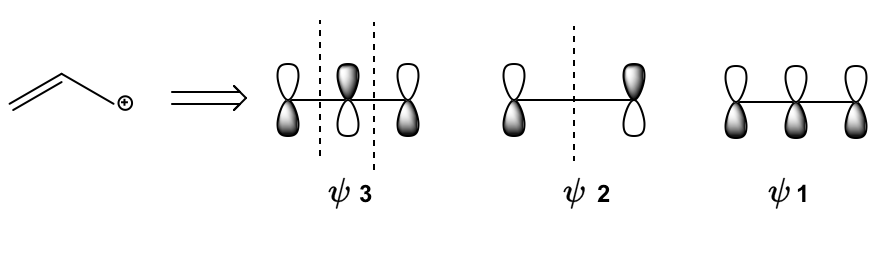Clutch Prep is now a part of Pearson
Ch. 16 - Conjugated SystemsWorksheetSee all chapters

# Orbital Diagram: 3-atoms- Allylic Ions

See all sections
Sections
Conjugation Chemistry
Stability of Conjugated Intermediates
Allylic Halogenation
Conjugated Hydrohalogenation (1,2 vs 1,4 addition)
Diels-Alder Reaction
Diels-Alder Forming Bridged Products
Diels-Alder Retrosynthesis
Molecular Orbital Theory
Drawing Atomic Orbitals
Drawing Molecular Orbitals
HOMO LUMO
Orbital Diagram: 3-atoms- Allylic Ions
Orbital Diagram: 5-atoms- Allylic Ions
Orbital Diagram: 6-atoms- 1,3,5-hexatriene
Orbital Diagram: Excited States
Pericyclic Reaction
Thermal Electrocyclic Reactions
Photochemical Electrocyclic Reactions
Cumulative Electrocyclic Problems
Sigmatropic Rearrangement
Cope Rearrangement
Claisen Rearrangement
Diene

Let's see what makes 3-atom conjugated systems unique from a molecular orbital perspective.

Concept #1

Transcript

Hey guys. In this video I'm going to show you how to draw molecular orbital diagrams for three atom conjugated systems, let's get started. So, guys because a 3 atom conjugated system is an odd number of atoms, what we're usually going to end up dealing with is an allylic position. Remember, that the allo position or the allylic position means it's the position next to a double bond and that's usually the case when you have an odd number of atoms because many times there's a double bond and then there's something next to the double bond, that's like the third atom, okay? Now, what we know about allylic positions from previous lessons is that allylic positions are able to resonate, remember? that the allylic position can resonate from one side of the allylic of the double bond to the other, okay? So, it turns out that that whole idea of resonating an allylic position can actually be explained through molecular orbitals, you already know how to explain it using resonance, you should be able to draw resonance arrows and be able to show how the allylic position can move from one side to the next but it actually turns out that the fundamental explanation of an allylic position reaction can actually be explained through molecular orbital series. So, that's what we're going to do right here, I'm going to show you guys how molecular orbitals explain the reactive positions of an allylic ion. So, here we go with the simplified LCAO model of a propanil ion and here what I've have drawn is a general propanil ion, basically you've got R3 atom conjugated system. So, we have three atomic orbitals like, let's just say A, B and C, right? And, what we see is that we have a double bond that we know that the double bond is going to contribute 1 pi electron for each atom. So, we have 1-2 and then we have this unknown ion, this unknown nonbonding orbital in position C and that is labeled as a question mark and the reason is because what I'm about to explain to you applies to any ion no matter what the identity of that question mark is whether it's empty, whether it's an empty orbital or whether it's a radical or whether it's a lone pair, it doesn't matter, what I want to teach you applies in all the situations and what the molecular orbital Theory would say about this molecule is that how would you, how would you structure the molecular orbitals? Well, it says that you would have first of all a molecular orbital with 0 node, one node and two nodes and that we would put those pi electrons in order of Aufbau principle. So, we would put the two electrons from that first double bond into side one, right? Which is the most stable of bonding orbital and then we would do, is do input whatever is left over whatever is here, whether it's 0 electrons, one electron or two electrons, they would go into side two, does that make sense so far? no matter, what the question mark is we know it's going into side two. Now, what is unique about side two, look at side two, notice that side two actually has a node at atom B, if this is atom A atom B and atom C, like we had before, we had A, B and C right? Notice there's a note at atom B, what does that mean? what that means is that regardless of the identity of the ion it can not react at position B, it can only react at either position A or at position C but because no electrons ever pass through atom B you will never find the allylic position reacting there. So what this does is it explains the theory behind the resonance structures that we've learned how to draw for so long. Remember, that an allylic position ion can resonate to the other side of the bond but it can never resonate to the middle, you've never seen that, for example, this let's say this is a positive charge, you can't move it to the middle, you can only switch places the double bond but you can't move it to the middle and the reason is because the molecular orbital theory states that there's no way that that ion can react at the middle carbon because that orbital doesn't even have any electrons, it can only react at the orbitals with electrons, which would either be orbital C in this case or orbital A in this case but never B, isn't that so cool? So what we're going to do next is an example.Example #1: Explaining Resonance through MO TheoryPractice: Consider the MO's of following allyl cation. Which of the following are HOMO and LUMO?